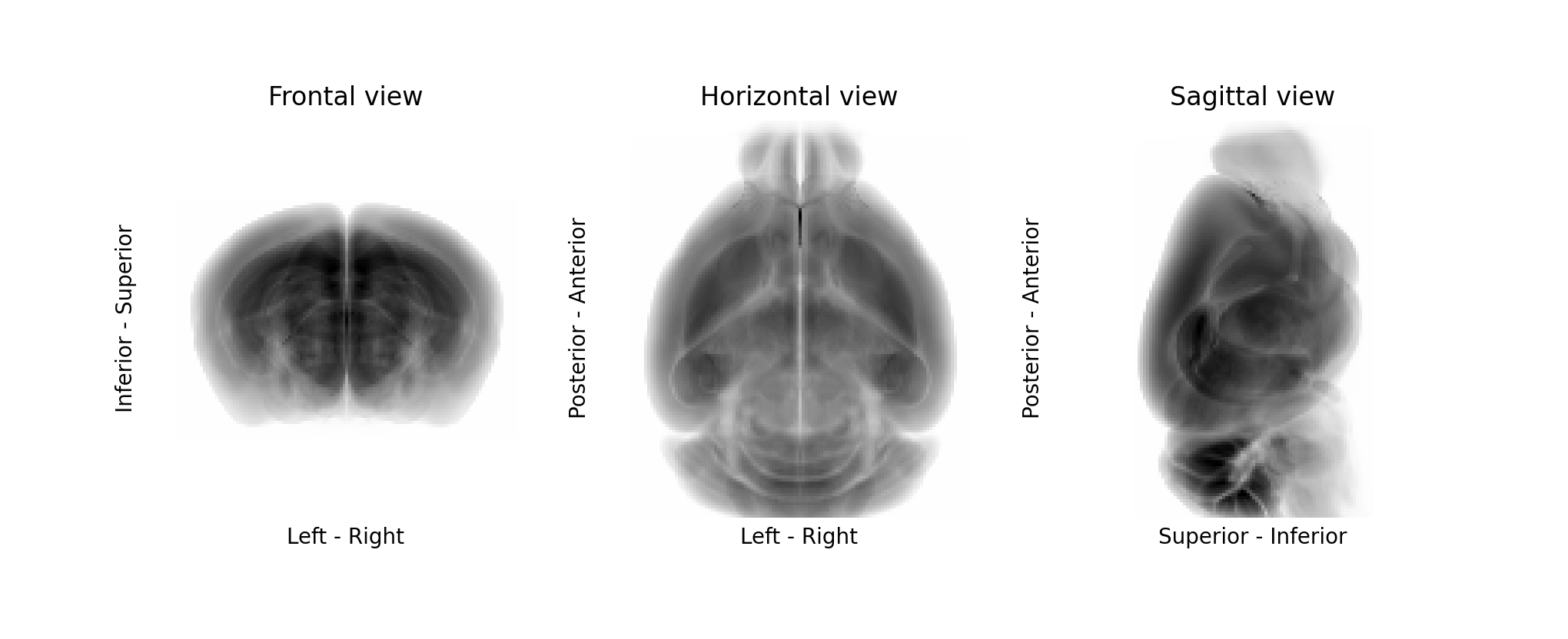# bg-space#

## Introduction#

Working with anatomical images, one often encounters the problem of matching the orientation of stacks with different conventions about axes orientation and order. Moreover, when multiple swaps and flips are involved, it can be annoying to map the same transformations to volumes and points (e.g., coordinates or meshes).

bg-space provides a neat way of defining an anatomical space, and of operating stacks and point transformations between spaces.

## Installation#

You can install bg-space with:

pip install bg-space


## Usage#

To define a new anatomical space, it is sufficient to give the directions of the stack origin position:

source_origin = ("Left", "Superior", "Anterior")
target_origin = ("Inferior", "Posterior", "Right")


A stack can be then easily transformed from the source to the target space:

import bg_space as bg
import numpy as np
stack = np.random.rand(3, 2, 4)

mapped_stack = bg.map_stack_to(source_origin, target_origin, stack)


The transformation is handled only with numpy index operations; i.e., no complex image affine transformations are applied. This is often useful as the preparatory step for starting any kind of image registration.

A shortened syntax can be used to define a space using initials of the origin directions:

mapped_stack = bg.map_stack_to("lsa", "ipr", stack)


Note

When you work with a stack, the origin is the upper left corner when you show the first element stack[0, :, :] with matplotlib or when you open the stack with ImageJ. The first dimension is the one that you are slicing, the second is the height of the image, and the third is the width of the image.

### The SpaceConvention class#

Sometimes, together with the stack, we have to move a set of points (cell coordinates, meshes, etc.). This introduces the additional complexity of keeping track, together with the axes swaps and flips, of the change of the origin offset.

To handle this situation, we can define a source space using the SpaceConvention class, specifying also the stack shape:

stack = np.random.rand(3, 2, 4)  # a stack in source space
annotations = np.array([[0, 0, 0], [2, 1, 3]])  # related point annotations

source_space = bg.SpaceConvention(target_origin, stack.shape)

mapped_stack = source_space.map_stack_to("ipr", stack)  # transform the stack
mapped_annotations = source_space.map_points_to("ipr", annotations)  # transform the points


The points are transformed through the generation of a transformation matrix. Finally, if we want to log this matrix (e.g., to reconstruct the full transformations sequence of a registration), we can get it:

target_space = bg.SpaceConvention("ipr", stack.shape)
transformation_matrix = SpaceConvention.transformation_matrix_to(target_space)
# equivalent to:
transformation_matrix = SpaceConvention.transformation_matrix_to("ipr", stack.shape)


The target get always be defined as a bg.SpaceConvention object, or a valid origin specification plus a shape (the shape is required only if axes flips are required).

### Matching space resolutions and offsets#

The SpaceConvention class can deal also with stack resampling/padding/cropping. This requires simply specifying values for resolutions and offsets when instantiating a SpaceConvention object. Once that is done, using SpaceConvention.transformation_matrix_to creating affine transformation matrices from one space to the other will be a piece of cake!

source_space = bgs.SpaceConvention("asl", resolution=(2, 1, 2), offset=(1, 0, 0))
target_space = bgs.SpaceConvention("sal", resolution=(1, 1, 1), offset=(0, 0, 2))
source_space.transformation_matrix_to(target_space)


Moreover, we can now use those space objects to resample stacks, and to generate stacks matching a target shape with the correct padding/cropping simply by specifying a target offset:

source_space = bgs.SpaceConvention("asl", resolution=(2, 1, 2), offset=(1, 0, 0))
target_space = bgs.SpaceConvention("asl", resolution=(1, 1, 1), shape=(5, 4, 2))  # we need a target shape
source_space.transformation_matrix_to(target_space, stack, to_target_shape=True)


### Easy iteration over projections#

Finally, another convenient feature of BG-Space is the possibility of iterating easily through the projections of the stack and generate section names and axes labels:

sc = bg.SpaceConvention("asl")  # origin for the stack to be plotted

for i, (plane, labels) in enumerate(zip(sc.sections, sc.axis_labels)):
axs[i].imshow(stack.mean(i))

axs[i].set_title(f"{plane.capitalize()} view")
axs[i].set_ylabel(labels)
axs[i].set_xlabel(labels)